CBSE Class 12 Sample Paper for 2018 Boards

Class 12
Solutions of Sample Papers and Past Year Papers - for Class 12 Boards

### If A = [2 1 1 1 0 1 0 2 -1] find the inverse of A using elementary row transformations and hence solve the following matrix equation XA = [1  0  1 ].

This is a question of CBSE Sample Paper - Class 12 - 2017/18.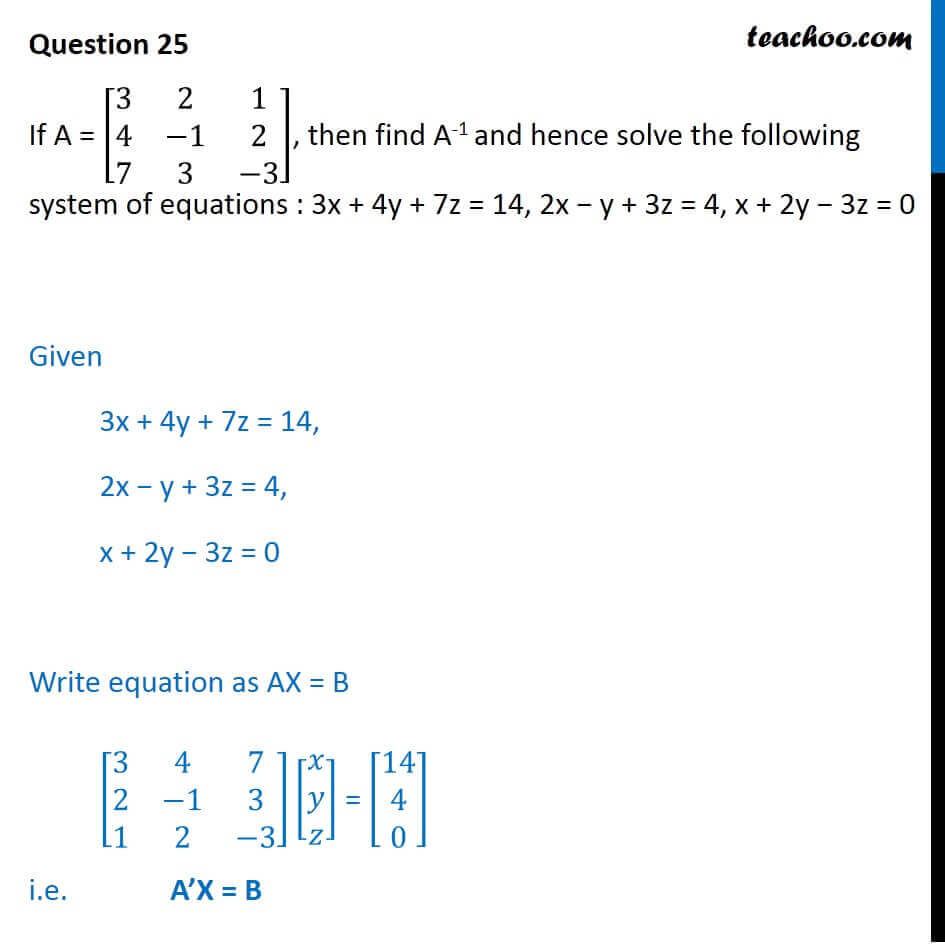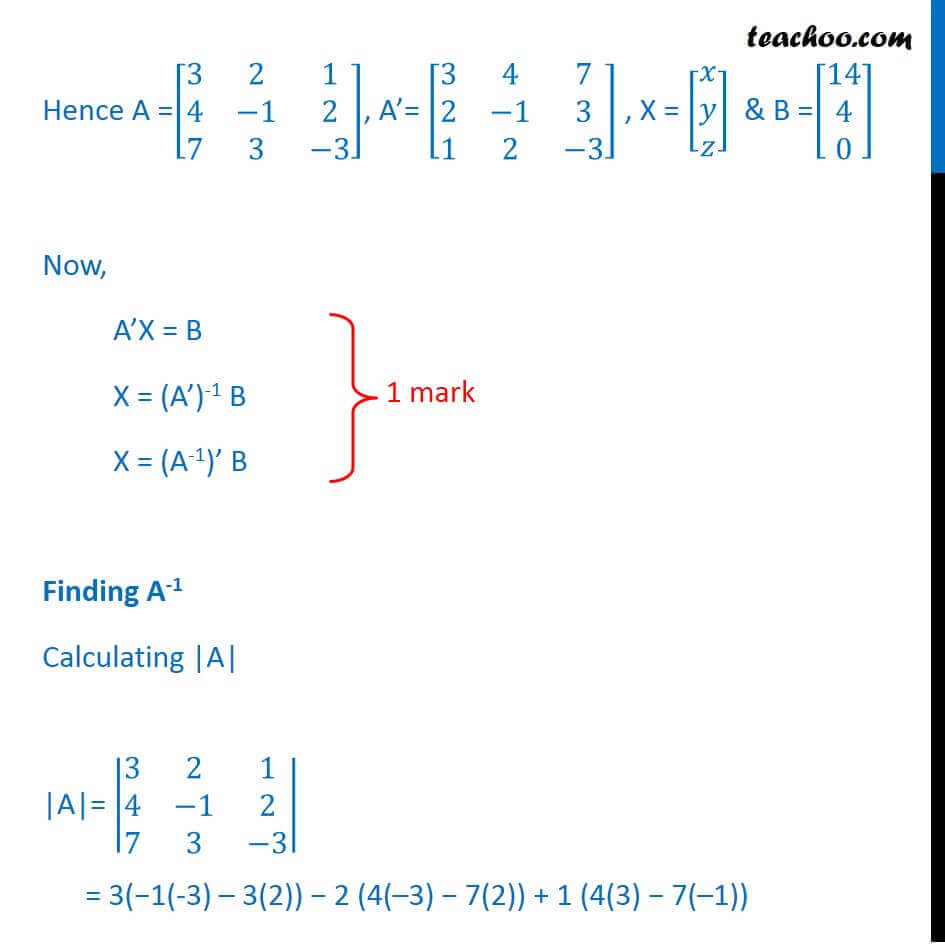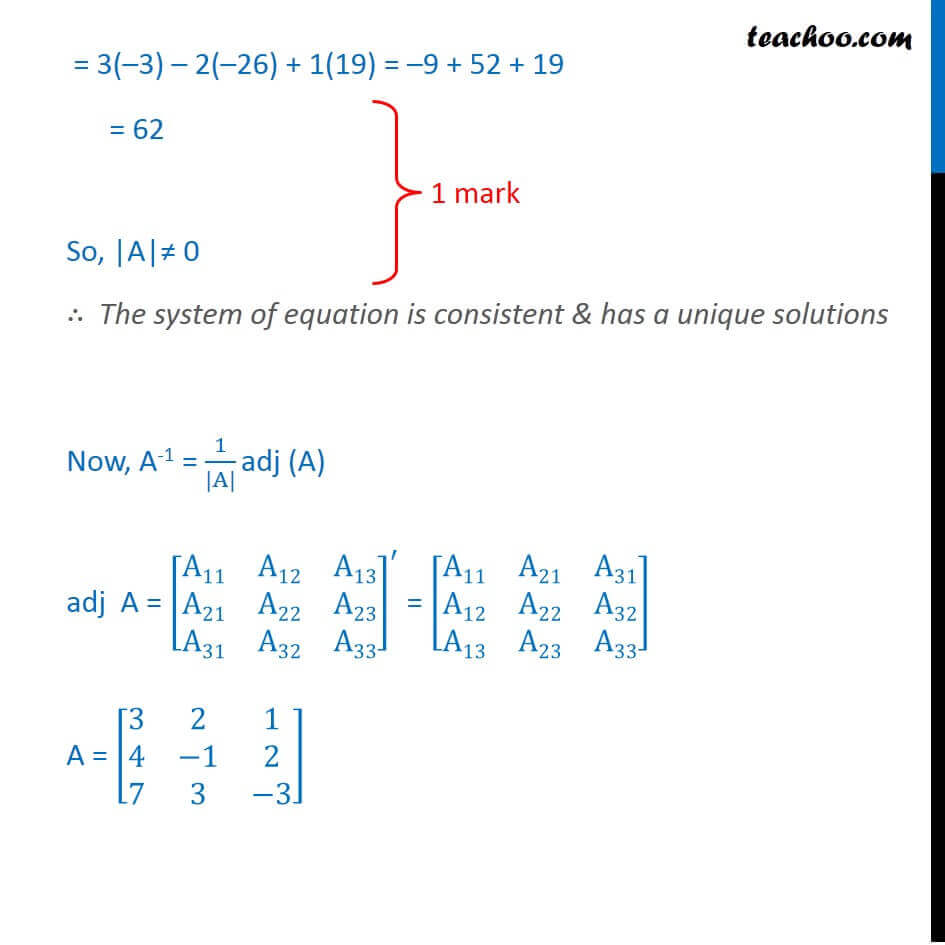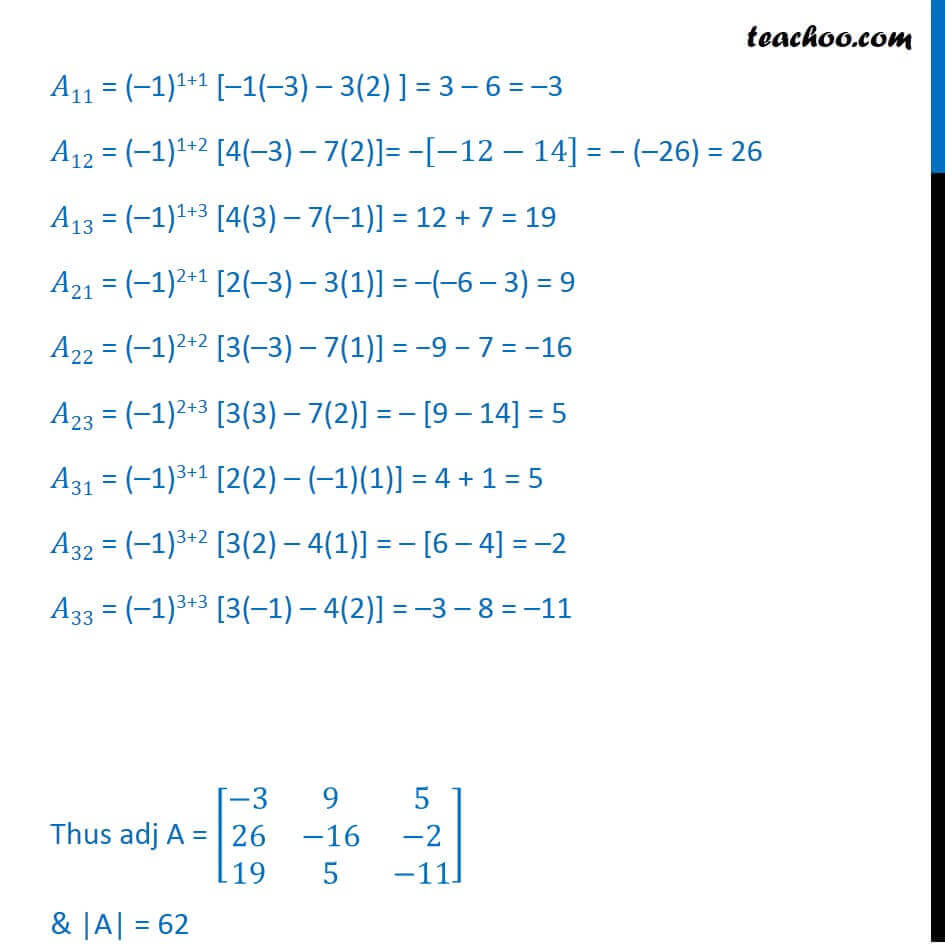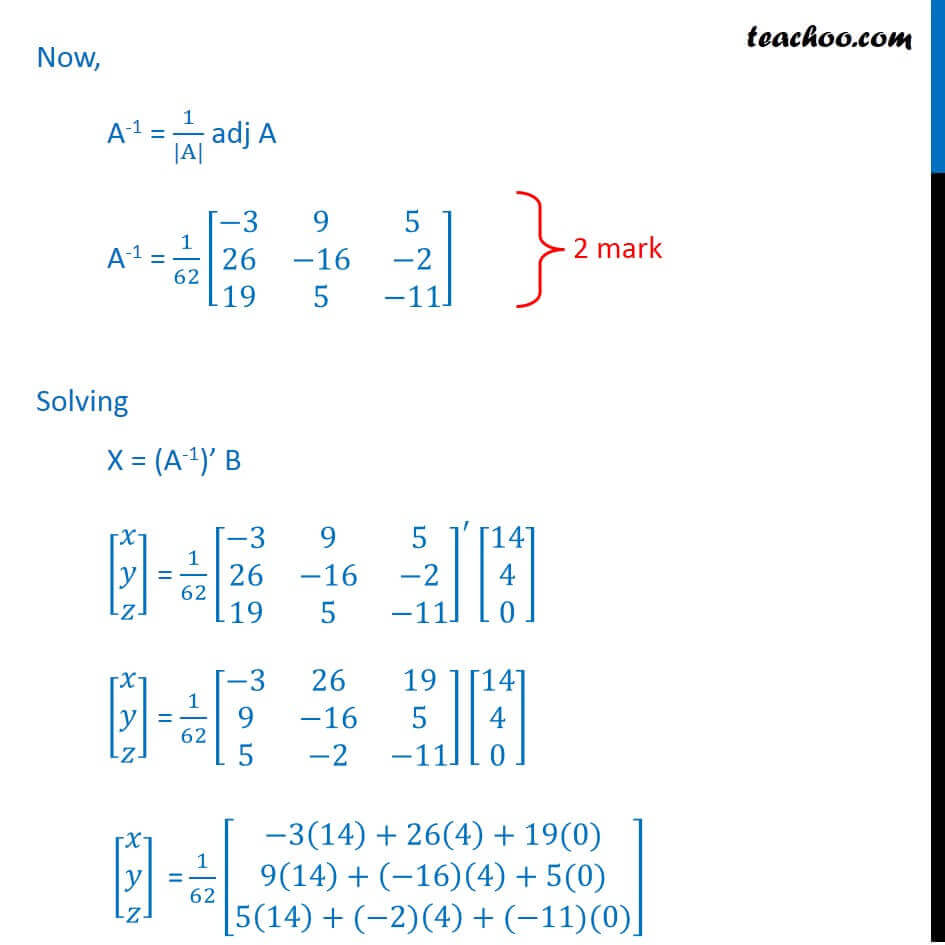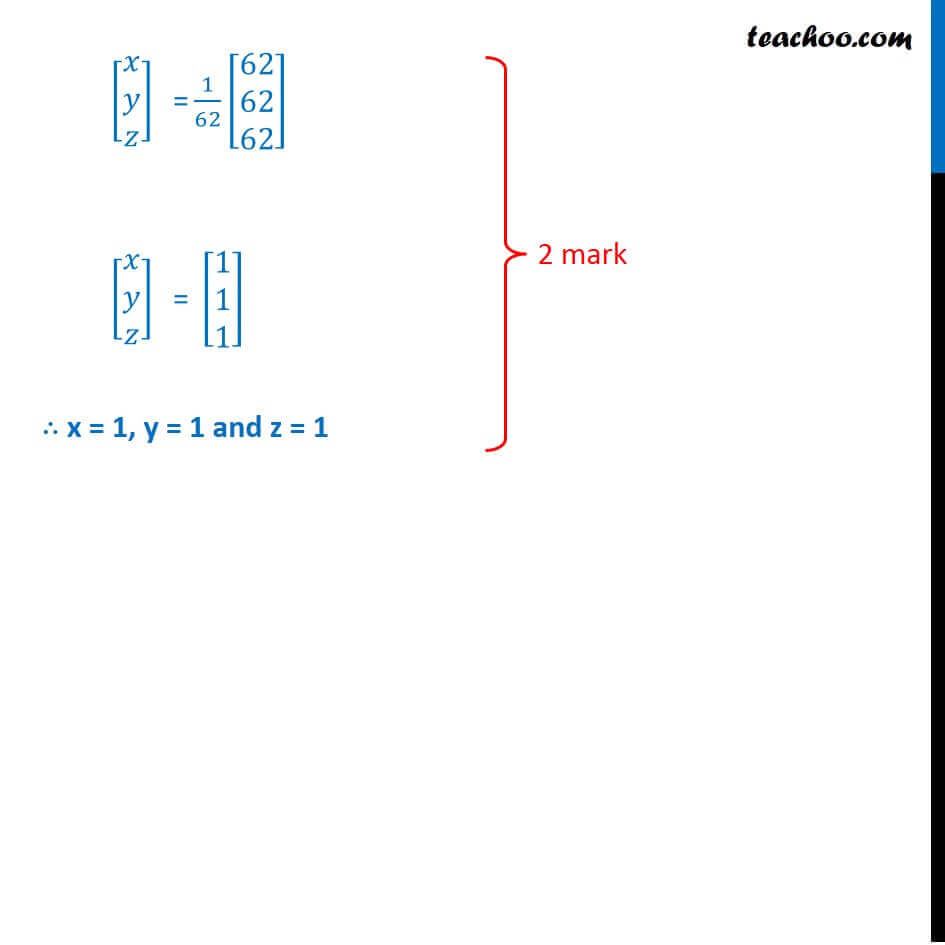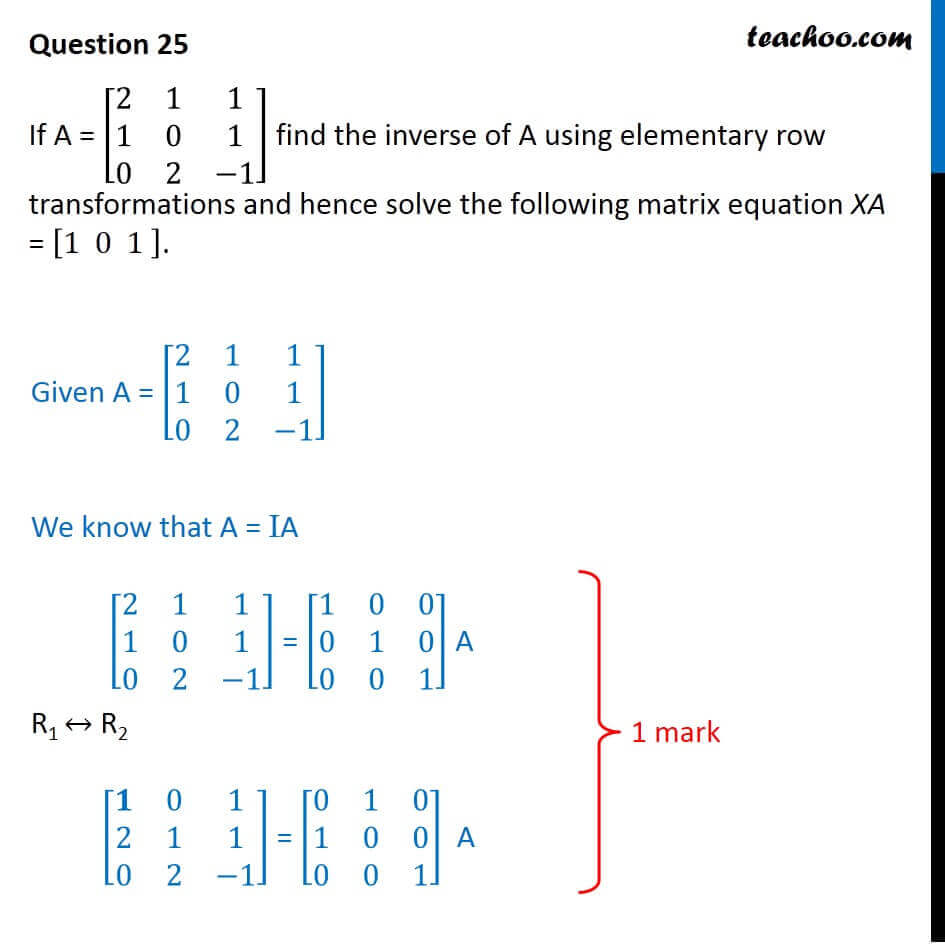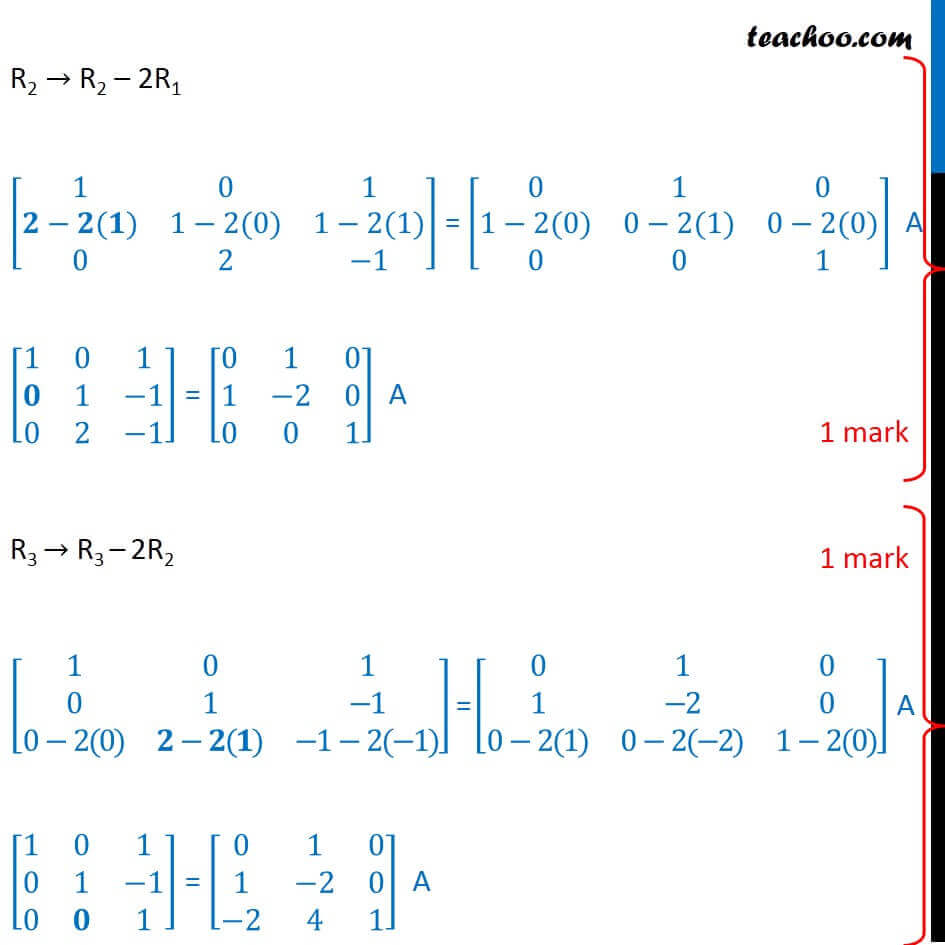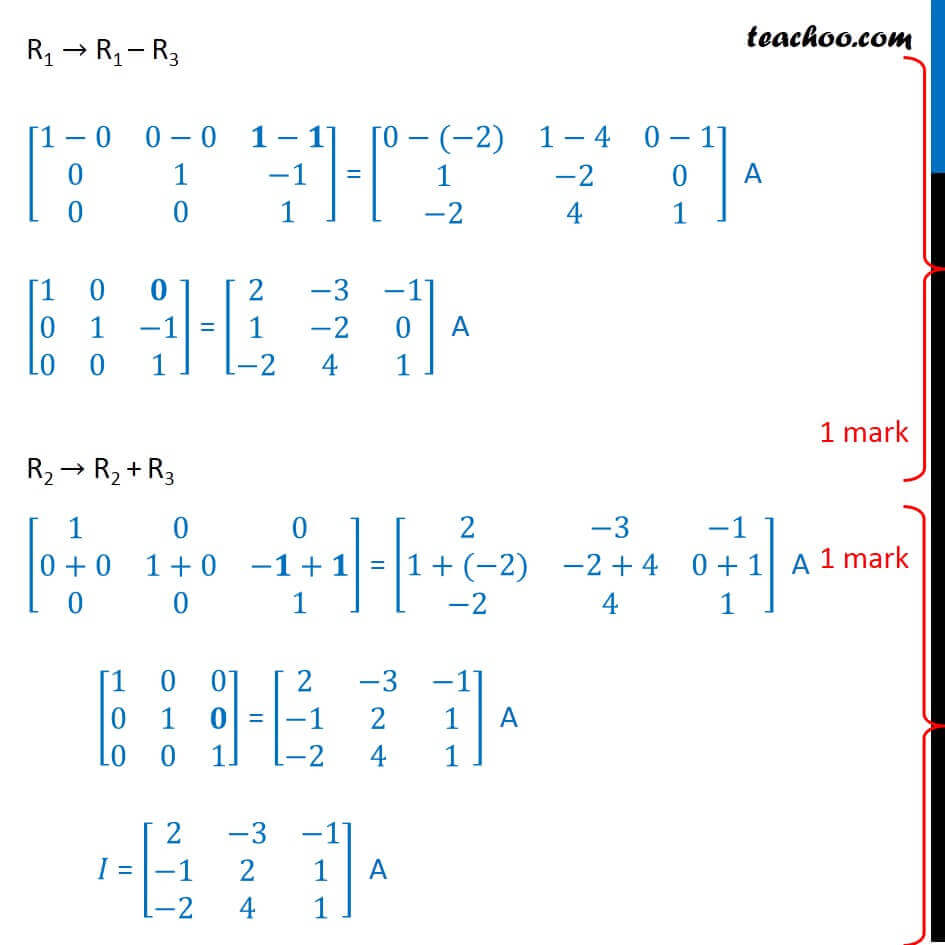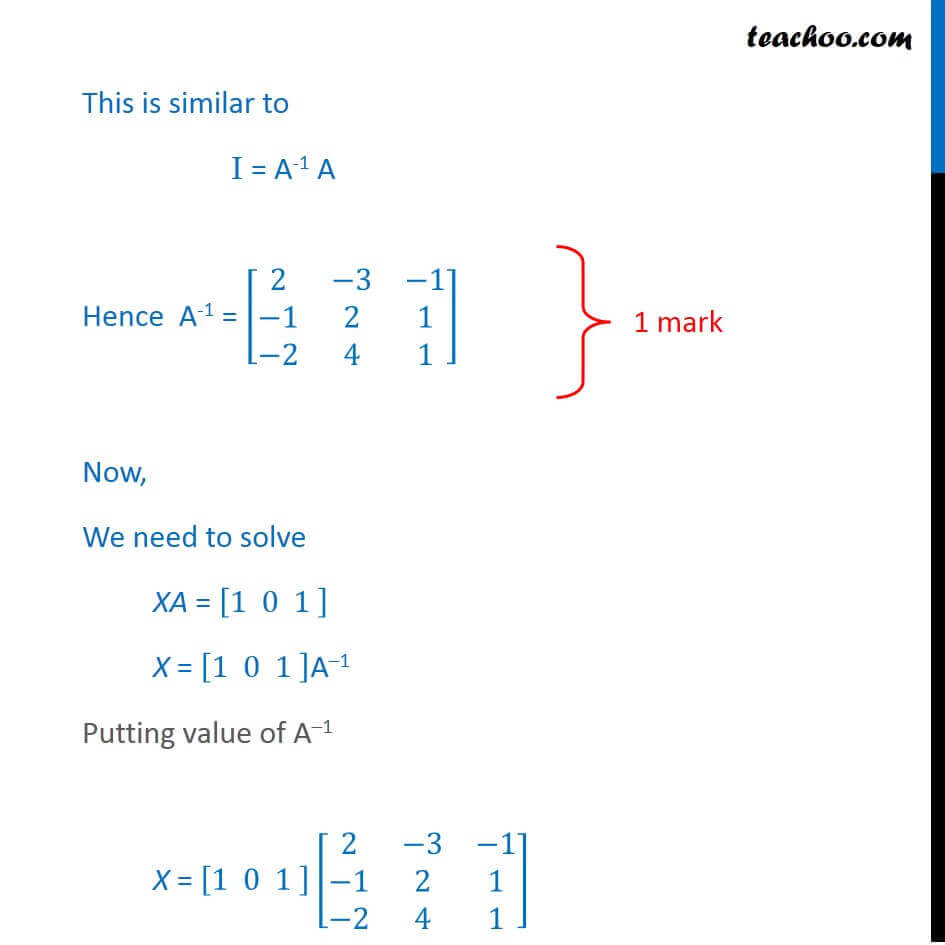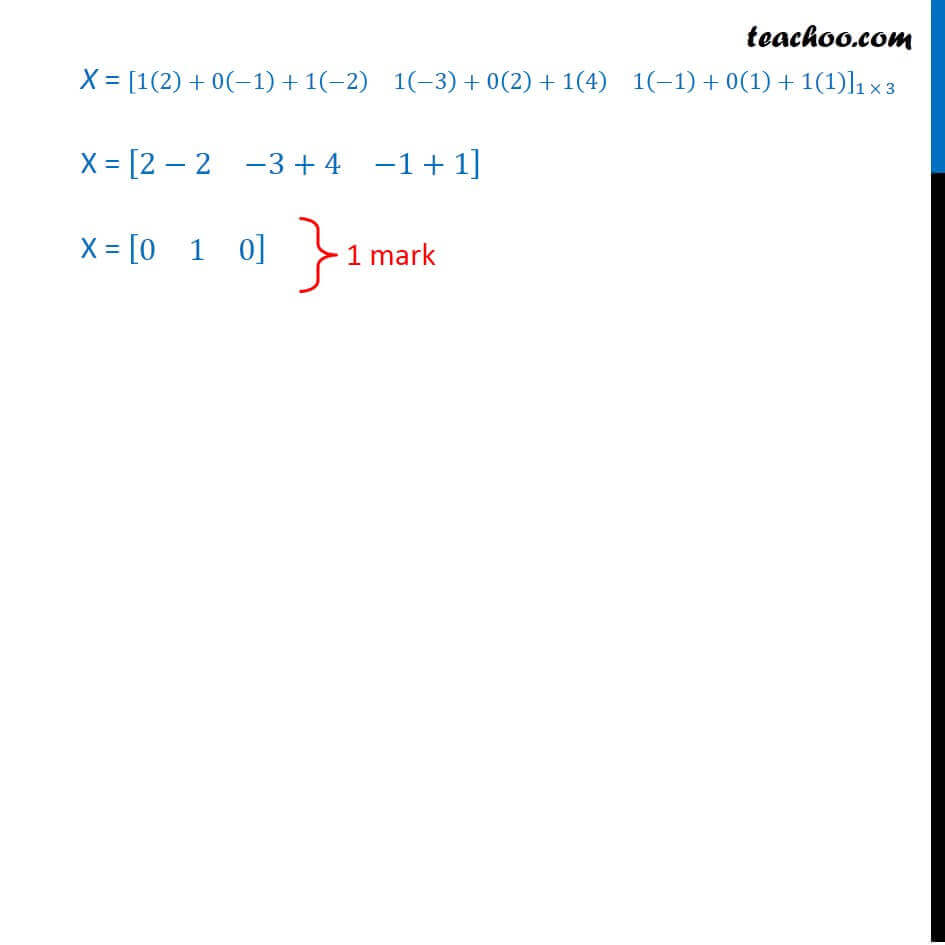Learn in your speed, with individual attention - Teachoo Maths 1-on-1 Class

### Transcript

Question 25 If A = [■8(3&2&1@4&−1&2@7&3&−3)], then find A-1 and hence solve the following system of equations : 3x + 4y + 7z = 14, 2x − y + 3z = 4, x + 2y − 3z = 0 Given 3x + 4y + 7z = 14, 2x − y + 3z = 4, x + 2y − 3z = 0 Write equation as AX = B [■8(3&4&7@2&−1&3@1&2&−3)][■8(𝑥@𝑦@𝑧)] = [■8(14@4@0)] i.e. A’X = B Hence A =[■8(3&2&1@4&−1&2@7&3&−3)], A’= [■8(3&4&7@2&−1&3@1&2&−3)] , X = [■8(𝑥@𝑦@𝑧)] & B =[■8(14@4@0)] Now, A’X = B X = (A’)-1 B X = (A-1)’ B Finding A-1 Calculating |A| |A|= |■8(3&2&1@4&−1&2@7&3&−3)| = 3(−1(-3) – 3(2)) − 2 (4(–3) − 7(2)) + 1 (4(3) − 7(–1)) = 3(–3) – 2(–26) + 1(19) = –9 + 52 + 19 = 62 So, |A|≠ 0 ∴ The system of equation is consistent & has a unique solutions Now, A-1 = 1/(|A|) adj (A) adj A = [■8(A11&A12&A13@A21&A22&A23@A31&A32&A33)]^′ = [■8(A11&A21&A31@A12&A22&A32@A13&A23&A33)] A = [■8(3&2&1@4&−1&2@7&3&−3)] 𝐴11 = (–1)1+1 [–1(–3) – 3(2) ] = 3 – 6 = –3 𝐴12 = (–1)1+2 [4(–3) – 7(2)]= −[−12−14] = − (–26) = 26 𝐴13 = (–1)1+3 [4(3) – 7(–1)] = 12 + 7 = 19 𝐴21 = (–1)2+1 [2(–3) – 3(1)] = –(–6 – 3) = 9 𝐴22 = (–1)2+2 [3(–3) – 7(1)] = −9 − 7 = −16 𝐴23 = (–1)2+3 [3(3) – 7(2)] = – [9 – 14] = 5 𝐴31 = (–1)3+1 [2(2) – (–1)(1)] = 4 + 1 = 5 𝐴32 = (–1)3+2 [3(2) – 4(1)] = – [6 – 4] = –2 𝐴33 = (–1)3+3 [3(–1) – 4(2)] = –3 – 8 = –11 Thus adj A = [■8(−3&9&5@26&−16&−2@19&5&−11)] & |A| = 62 Now, A-1 = 1/(|A|) adj A A-1 = 1/62 [■8(−3&9&5@26&−16&−2@19&5&−11)] Solving X = (A-1)’ B [■8(𝑥@𝑦@𝑧)] = 1/62 [■8(−3&9&5@26&−16&−2@19&5&−11)]^′ [■8(14@4@0)] [■8(𝑥@𝑦@𝑧)] = 1/62 [■8(−3&26&19@9&−16&5@5&−2&−11)][■8(14@4@0)] " " [■8(𝑥@𝑦@𝑧)]" =" 1/62 [█(−3(14)+26(4)+19(0)@9(14)+(−16)(4)+5(0)@5(14)+(−2)(4)+(−11)(0))] " " [■8(𝑥@𝑦@𝑧)]" =" 1/62 [■8(62@62@62)] " " [■8(𝑥@𝑦@𝑧)]" =" [■8(1@1@1)] "∴ x = 1, y = 1 and z = 1 " Question 25 If A = [■8(2&1&1@1&0&1@0&2&−1)] find the inverse of A using elementary row transformations and hence solve the following matrix equation XA = [1 0 1 ]. Given A = [■8(2&1&1@1&0&1@0&2&−1)] We know that A = IA [■8(2&1&1@1&0&1@0&2&−1)] = [■8(1&0&0@0&1&0@0&0&1)] A R1 ↔ R2 [■8(𝟏&0&1@2&1&1@0&2&−1)] = [■8(0&1&0@1&0&0@0&0&1)] A R2 → R2 – 2R1 [■8(1&0&1@𝟐−𝟐(𝟏)&1−2(0)&1−2(1)@0&2&−1)] = [■8(0&1&0@1−2(0)&0−2(1)&0−2(0)@0&0&1)] A [■8(1&0&1@𝟎&1&−1@0&2&−1)] = [■8(0&1&0@1&−2&0@0&0&1)] A R3 → R3 – 2R2 [■8(1&0&1@0&1&−1@0−2(0)&𝟐−𝟐(𝟏)&−1−2(−1))] = [■8(0&1&0@1&−2&0@0−2(1)&0−2(−2)&1−2(0))] A [■8(1&0&1@0&1&−1@0&𝟎&1)] = [■8(0&1&0@1&−2&0@−2&4&1)] A R1 → R1 – R3 [■8(1−0&0−0&𝟏−𝟏@0&1&−1@0&0&1)] = [■8(0−(−2)&1−4&0−1@1&−2&0@−2&4&1)] A [■8(1&0&𝟎@0&1&−1@0&0&1)] = [■8(2&−3&−1@1&−2&0@−2&4&1)] A R2 → R2 + R3 [■8(1&0&0@0+0&1+0&−𝟏+𝟏@0&0&1)] = [■8(2&−3&−1@1+(−2)&−2+4&0+1@−2&4&1)] A [■8(1&0&0@0&1&𝟎@0&0&1)] = [■8(2&−3&−1@−1&2&1@−2&4&1)] A 𝐼 = [■8(2&−3&−1@−1&2&1@−2&4&1)] A R1 → R1 – R3 [■8(1−0&0−0&𝟏−𝟏@0&1&−1@0&0&1)] = [■8(0−(−2)&1−4&0−1@1&−2&0@−2&4&1)] A [■8(1&0&𝟎@0&1&−1@0&0&1)] = [■8(2&−3&−1@1&−2&0@−2&4&1)] A R2 → R2 + R3 [■8(1&0&0@0+0&1+0&−𝟏+𝟏@0&0&1)] = [■8(2&−3&−1@1+(−2)&−2+4&0+1@−2&4&1)] A [■8(1&0&0@0&1&𝟎@0&0&1)] = [■8(2&−3&−1@−1&2&1@−2&4&1)] A 𝐼 = [■8(2&−3&−1@−1&2&1@−2&4&1)] A This is similar to I = A-1 A Hence A-1 = [■8(2&−3&−1@−1&2&1@−2&4&1)] Now, We need to solve XA = [1 0 1 ] X = [1 0 1 ]A–1 Putting value of A–1 X = [1 0 1 ][■8(2&−3&−1@−1&2&1@−2&4&1)] X = [1 0 1 ]_(1 × 3) [■8(2&−3&−1@−1&2&1@−2&4&1)]_(3 × 3) X = [■8(1(2)+0(−1)+1(−2)&1(−3)+0(2)+1(4)&1(−1)+0(1)+1(1))]_(1 × 3) X = [■8(2−2&−3+4&−1+1)] X = [■8(0&1&0)]